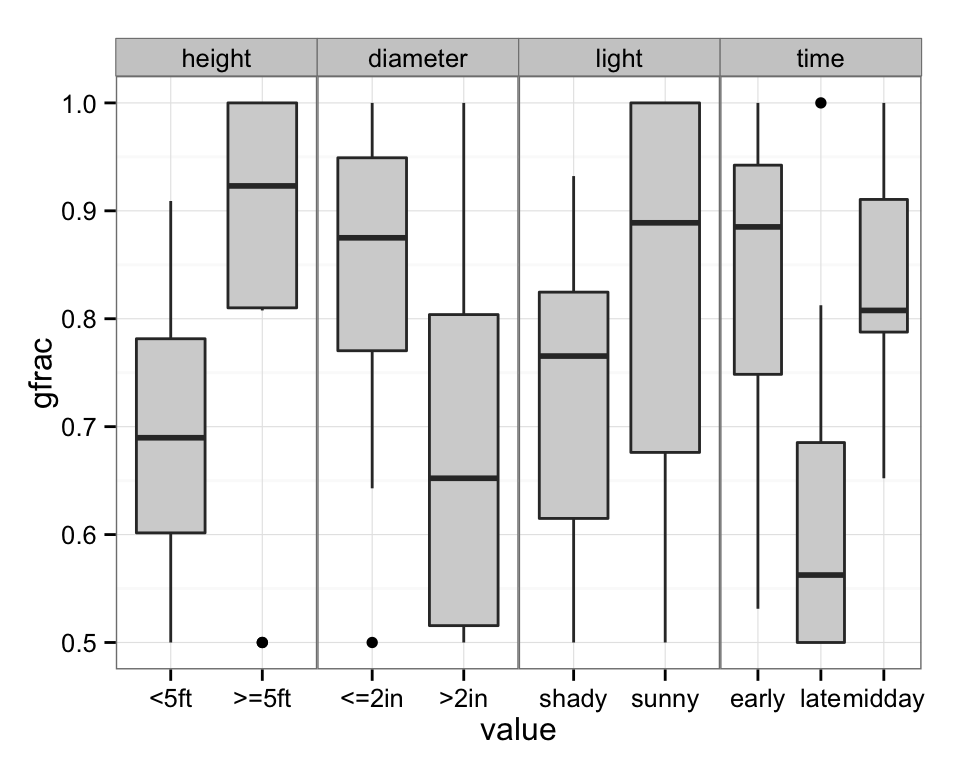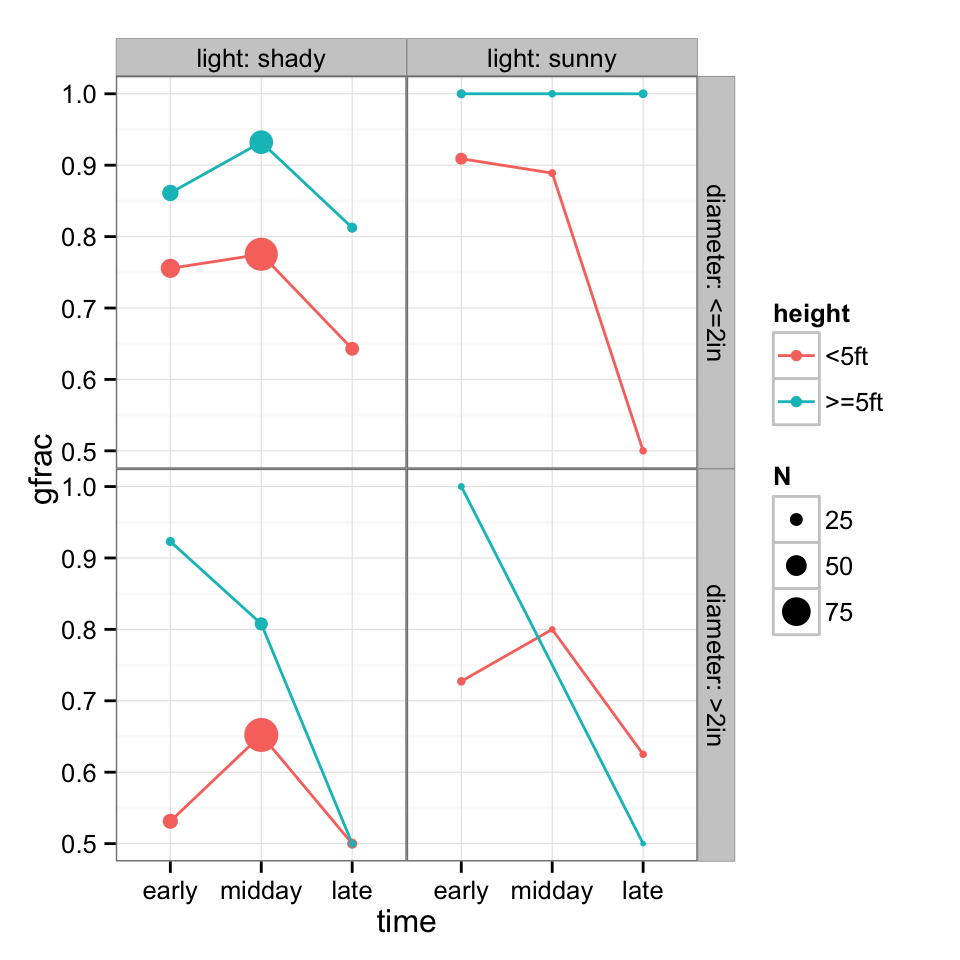In this exercise we’ll fit a simple model and apply a variety of model diagnostics to it. Some may be very familiar …

``````library(arm) ## for sim()
library(descr)  ## for LogRegR2
require("reshape2")
## graphics prettiness
library("ggplot2")
theme_set(theme_bw())
library("grid")
zmargin <- theme(panel.margin=unit(0,"lines"))``````

Data on lizard perching behaviour, from the `brglm` package (and before that from McCullagh and Nelder (McCullagh and Nelder 1989), ultimately from Schoener (1970)).

``````lizards <- read.csv("data/lizards.csv")
## adjust factor levels to a sensible order
lizards\$time <- factor(lizards\$time,
levels=c("early","midday","late"))``````

A quick look at the data: response is fraction of Anolis grahami lizards found on perches in particular conditions. Plot univariate responses:A more conventional plot:

``````(g1 <- ggplot(lizards,
aes(x=time,y=gfrac,colour=height))+
geom_point(aes(size=N))+
geom_line(aes(group=height))+
facet_grid(diameter~light,labeller=label_both)+zmargin)``````Fit a basic (additive: no interactions) binomial GLM:

``````m1 <- glm(gfrac~time+height+light+diameter,
weights=N,
family="binomial",
data=lizards)``````

Standard R diagnostic plots (fitted vs. residual, scale-location, Q-Q, influence):

``````op <- par(mfrow=c(2,2))  ## 2x2 subplot grid
plot(m1)``````